Damping Factor

The damping factor refers to the damping ratio for each frequency. It makes it possible to obtain the damping ratio of the target frequency and affects the selection of materials together with the natural frequency.

The value of the damping factor is represented in percent. The larger (smaller) the damping factor is, the quicker (slower) the frequency dampens. The FFT analyzer makes it easier, for each resonance frequency, to obtain the damping factor as the inverse of the ratio of the -3dB frequency width to the resonance frequency.

Decibel (dB)

Voltage, sound pressure, etc. are often expressed in dB (decibels). The dB notation uses the common logarithms (log10).

We learned the following logarithm rules in high school math class: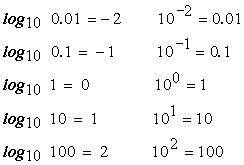and the following logarithmic operation rules: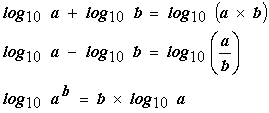dB (decibel) is defied as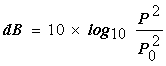where P0 is the reference level with which a voltage of 1V and a sound pressure of 20μPa (Pascal) are used. dB expresses the ratio of square or power. If P is the same as the reference level, P2/P02=1 or 0dB because log101=0.  With 0dB=1 (P0=1) in mind, let us calculate 10 times in dB.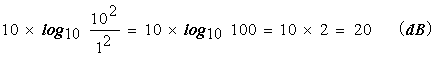10 times corresponds to 20dB. Since it is troublesome to square the ratio each time, 20log is made using a previous logarithmic operation rule.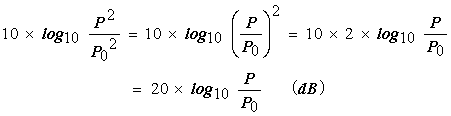This eliminates the need to square the ratio resulting in simple calculation. This notation may not be familiar in mathematics. When the antilogarithm (the number subject to logarithmic operation, a and b above) is decupled or multiplied by 10, the result is 20dB (because 10=101). When the antilogarithm is multiplied by 1/10, the result is -20dB (because 1/10=10-1).

Energy Spectrum Density

The finite impulse energy (by striking method, etc.), standardized in terms of energy.

The energy spectrum density is calculated by multiplying the power spectrum density by the sampling time (window length, T=1/⊿f).

Fourier Transform

When time function x (t) defined over variable range -T/2 <= t <= T/2 is represented by expression (2) using the Fourier coefficient in expression (1), the function x (t) can be recognized as a periodic function which repeats the same waveform outside the variable range as the one inside it according to the periodicity of the complex exponential function. Therefore, the periodic function x (t) which repeats the same waveform with a period of T is represented by the Fourier series.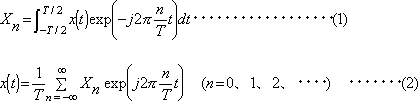In order to obtain the limit of expressions (1) and (2) when the time section length (T) is an infinite, which defines the time function x (t), the following transformation (expression (3)) is applied to the two expressions. In this case, the summation in expression (2) is replaced with integral, resulting in a transformation pair given by expressions (4) and (5).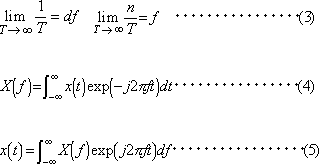Expression (4) denotes the Fourier transform of x (t) and expression (5) the inverse Fourier transform of X (f). This pair in expressions is referred to as the Fourier transform pair or Fourier integral pair. X (f), a function of frequency f, is also referred to as the complex amplitude. As shown by expressions (4) and (5), the Fourier transform obtains the corresponding frequency function from a time function, and the inverse Fourier transform obtains the corresponding time function from a frequency function.

The following transformation pair may be used in some cases, which defines the integral section for the Fourier transform as a semi-infinite section assuming that the start point of time function x (t) is the origin of the time axis and that x (t) is zero in the section with t<0.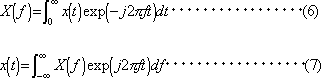When complex function X (f) of the frequency defined by expression (4) is now represented by expression(8), the components of frequency f forming time function x (t) are represented by expression (9) from the definition of expression (5).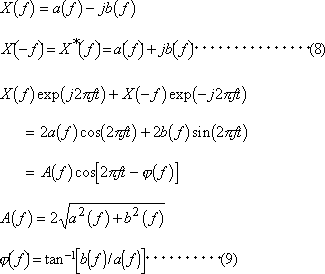That is, the amplitude of the component of frequency f is equal to 2|X(f) | and its phase is equal to declination ∠X (f) of
X (f).  Thus, the amplitude and phase of the component of time function x (t) can be obtained from complex number X (f), and therefore X (f) is referred to as the frequency spectrum of x (t).

The Fourier coefficient obtained by expression (1) has its value only at frequency n/T and is always zero at other frequencies, and therefore it is referred to as line spectrum. On the other hand, X (f) of expression (4) is a continuous function of the frequency, and therefore it is referred to as continuous spectrum.

Frequency-Axis Differential/Integral

Frequency differential is performed by multiplying the power spectrum and the frequency response function by (jω)n.
(j is the imaginary number unit and ω=2πf.)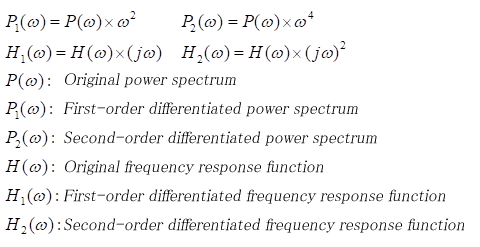(Note 1) In the power spectrum, the first-order differential is the square of ω and the second-order differential is the fourth power of ω because they are power values.
(Note 2) Since the frequency response function is a complex arithmetic operation, the imaginary units j are also multiplied.

The integration operation on the frequency axis is performed by dividing by (ω)n for the power spectrum and (jω)n for the frequency response function.
(j is an imaginary unit, ω＝2πｆ)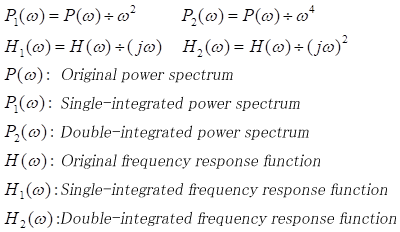(Note 1) In the power spectrum, the single integration is the square of ω and the double integration is the fourth power of ω, because they are power values.
(Note 2) Since the frequency response function is a complex arithmetic operation, the imaginary units j are also divided.

Frequency Resolution

The frequency resolution is equal to the frequency range divided by the number of FFT lines (analysis data length HREF_FRAME_LEN/2.56).

At the time of zoom analysis, the frequency resolution is equal to 1/800 times (stop frequency - start frequency).

Frequency Response Function

The frequency response function denotes the relationship between the input and the output of an electrical system or structural vibration transmission system. It is represented by the ratio of the Fourier spectrum of the input, A (f), to the Fourier spectrum of the output, B (f).

Therefore, the frequency response function, H(f), can be represented by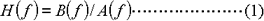This FFT analyzer calculates H (f) by multiplying each of the denominator and numerator on the right side by A*(f), complex conjugate of A (f), using the following expression.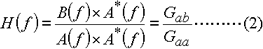The denominator, A (f) x A*(f), is the power spectrum of A (f), and the numerator, B (f) x A*(f), is the cross spectrum of A (f) and B (f). Therefore, the frequency response function, H (f), can be obtained by dividing the cross spectrum of the input and output by the power spectrum of the input.

The frequency response function can also be estimated by expression (3).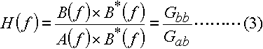The transfer function estimated by expression (2) is referred to as H1 and the one estimated by expression (3) as H2.

 H1: Gab/Gaa If output signal b (t) contains much external noise, random error can be minimized by averaging.  A non-linear system can be linearized by averaging (approximation with method of least squares) using a random signal as an input signal.

 H2: Gbb/Gab If output signal a (t) contains much external noise, random error can be minimized by averaging. If leakage error is assumed at the resonance point, bias error can be reduced.

When the true frequency response function is Ht(f), if both input and output contain much noise, the following relationship results (on the premise that the system is a linear system).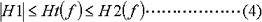The phase of H1(f),H2(f) is equal to the phase of cross spectrum Gab.

The relationship with coherence function HREF_COH is represented by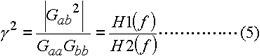which is the ratio of H1 to H2. When the ratio of the power spectrum for input and output (transfer characteristic) is |Ha(f)|2,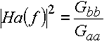Therefore,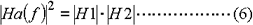If you apply logarithm to both sides of expression (6),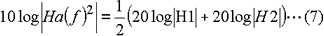Expression (7) indicates that the average of the logarithmic value of the gain of H1 and H2 is equal to the logarithmic value of true frequency response function Ha.

The frequency response function can be represented by the gain and phase characteristics. The gain characteristic indicates how the amplitude varies when a signal passes through the system. The X-axis denotes the frequency and the Y-axis decibel based on 20 log10 H (f). The phase characteristic indicates the phase lead or lag between the input signal and the output signal. The X-axis denotes the frequency and the Y-axis the angle in degree or radian